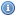# Mathematical Logic and Set Theory Books, Documents, and Lectures

 Title Year DescriptionA Problem Course in Mathematical Logic 2003A text indended to serve as an introduction to mathematical logic for undergraduates with some mathematical sophistication. It supplies definitions, statements of results, and problems, along with some explanations, examples, and hints.Logic For Everyone 1994This book covers all of the basic material in the propositional and predicate calculus. Applications include non-standard models obtained by the means of compactness theorem and the generation of weak ultrawords and ultralogic operator.Mathematical Logic - An Introduction 2006These notes introduce mathematical logic and include an extensive section on the history of logic